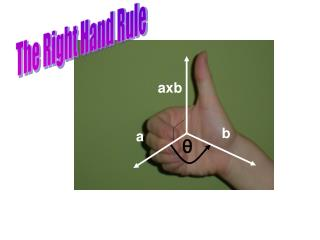DownloadDownload Presentationaxb

# axb

Télécharger la présentation## axb

- - - - - - - - - - - - - - - - - - - - - - - - - - - E N D - - - - - - - - - - - - - - - - - - - - - - - - - - -
##### Presentation Transcript

1. The Right Hand Rule axb b a

2. Properties of the Cross Product • axb = -bxa • (ca)xb = c(axb) = ax(cb) • ax(b+c) = axb + axc • (a+b)xc = axc + bxc • a.(bxc) = (axb).c • ax(bxc) =(a.c)b-(a.b)c

3. The so-called scalar triplea.(bxc) But recall that the components of (bxc) come from 2x2 determinants

4. a.(bxc) A-ha! We have a quick way to compute this!

5. V = |bxc| |a||cos( )|=|a.(bxc)| The Geometric InterpretationVolume of a parallelpiped bxc a h c b Volume = (Area of Base)*(height)

6. Q. How can we use the cross product to determine if 3 vectors are coplanar (lie in the same plane)? • Determine if the volume of the • resulting parallelpiped is nonzero

7. Example Do the following points lie in the same plane? A=(1,-1,2) B=(2,0,1) C=(3,2,0) D=(5,4,2)